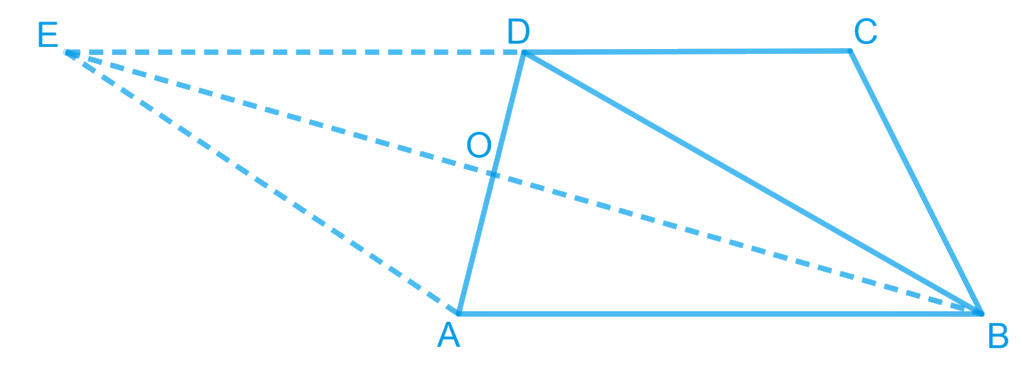# Ex.9.3 Q12 Areas of Parallelograms and Triangles Solution - NCERT Maths Class 9

## Question

A villager Itwaari has a plot of land of the shape of a quadrilateral. The Gram Panchayat of the village decided to take over some portion of his plot from one of the corners to construct a Health Centre. Itwaari agrees to the above proposal with the condition that he should be given equal amount of land in lieu of his land adjoining his plot so as to form a triangular plot. Explain how this proposal will be implemented.

Video Solution
Areas Of Parallelograms And Triangles
Ex 9.3 | Question 12

## Text Solution

What is known?

Itwaari has a plot of land of the shape of a quadrilateral and some portion of his plot Gram Panchayat use to construct Health Centre. He should be given equal amount of land in lieu of his land adjoining his plot so as to form a triangular plot.

What is unknown?

How this proposal will be implemented.

Reasoning:

Join diagonal $$BD$$ and draw a line parallel to $$BD$$ through point A.Let it meet the extended side $$CD$$ of $$ABCD$$ at point $$E$$.Join $$BE$$ and $$AD$$. Let them intersect each other at O. We can use theorem for triangles $$DEB$$ and $$DAB$$, if two triangles are on same base and between same pair of parallel lines then both will have equal area. Now we can subtract area of triangle DOB from both sides to get the required result.

Steps:Let quadrilateral $$ABCD$$ be the original shape of the field.

The proposal may be implemented as follows.

Join diagonal $$BD$$ and draw a line parallel to $$BD$$ through point $$A$$.

Let it meet the extended side $$CD$$ of $$ABCD$$ at point $$E$$.

Join $$BE$$ and $$AD$$. Let them intersect each other at $$O$$.

Then, portion $$\Delta AOB$$ can be cut from the original field so that the new shape of the field will be . (See figure).

We have to prove that the area of $$\Delta AOB$$ (portion that was cut so as to construct Health Centre) is equal to the area of $$\Delta DEO$$ (portion added to the field so as to make the area of the new field so formed equal to the area of the original field).

It can be observed that $$ΔDEB$$ and $$ΔDAB$$ lie on the same base $$BD$$ and are between the same parallels $$BD$$ and $$AE$$.

According to Theorem 9.2 : Two triangles on the same base (or equal bases) and between the same parallels are equal in area.

\begin{align}{\text{ Area }}(\Delta {{DEB}})&\!\!= \!\!{\text{ Area }}(\Delta {{DAB}})\\\left[ \begin{array}{l}{\text{Area }}(\Delta {{DEB}}) - \\{\text{ Area }}(\Delta {{DOB}})\end{array} \!\right] &\!\!=\!\!\left[ \begin{array}{l}{\text{Area }}(\Delta {{DAB}}) - \\ {\text{Area }}(\Delta {{DOB}})\end{array}\!\right]\\{\text{Area}}\left( {\Delta {{DEO}}} \right)&\!\!=\!\! {\text{Area}}\left( {\Delta {{AOB}}} \right)\end{align}

Video Solution
Areas Of Parallelograms And Triangles
Ex 9.3 | Question 12
Learn from the best math teachers and top your exams

• Live one on one classroom and doubt clearing
• Practice worksheets in and after class for conceptual clarity
• Personalized curriculum to keep up with school